# Transfinite Cardinal Arithmetic with Wolfram|Alpha

September 10, 2010 —Comments Off

The concept of infinity has been fraught with paradox since antiquity. For this reason, Aristotle sought to banish it from his physics, claiming that there were no actual infinities in nature—only potential infinities. Over a millennium later, medieval scholars offered the following example when asked why infinity was forbidden.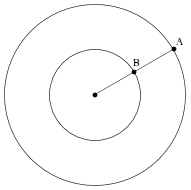Imagine two concentric circles. Each circle contains infinitely many points along its circumference, but since the outer circle has a greater circumference, it has more points than the inner circle. Now take any point A on the outer circle, and draw a line from A to the circle’s center. This line must intersect some point B on the circumference of the inner circle. Hence, for every point A on the outer circle, there is a corresponding point B on the inner circle, and vice versa. Therefore, both circles must have the same number of points, despite the fact that the outer circle appears to have more points than the inner circle.

Many thinkers tried to unravel this apparent paradox, including Galileo Galilei, but it wasn’t until the late 1800s that Georg Cantor brought it to a successful resolution. Cantor’s idea was simple: infinite sets of objects are so far removed from our everyday experience that they may follow rules that seem counterintuitive. For Cantor, it simply must be accepted that the inner and outer circles have the same number of points—our intuition that the outer circle has more points, according to Cantor, is just wrong.

Instead, he focused on the fact that there exists a one-to-one correspondence between the points on the outer circle and the points on the inner circle. Cantor’s insight was to use this idea as the definition for determining whether or not two sets of objects are the same size. With this definition, we no longer need to use our intuition to test whether or not sets of points have different sizes; we only have to check whether or not there exists a one-to-one correspondence between the given pair of sets. If there is no one-to-one correspondence then, according to Cantor, one of the two sets must be larger than the other.

Cantor’s definition tamed infinity. It provided a rigorous method by which infinite sets could be compared. He used this idea to prove that there are just as many integers as even integers, since the function f(x)=2x provides the required one-to-one correspondence. He showed that there are just as many lattice points on the plane as there are integers. And Cantor even proved that there are as many rational numbers as there are integers.

But not all infinite sets have the same size as the set of integers. Cantor was able to show, using his now-famous diagonal argument, that there are more real numbers than integers. This led him to introduce a distinction between countable and uncountable infinities. Sets that have the same size as the set of integers are said to be countable, while sets that are larger than the set of integers are uncountable. He also introduced the notion of a transfinite cardinal number to quantify the different magnitudes of infinite sets. The smallest transfinite cardinal is ℵ0 (pronounced “aleph-zero”). It represents the size of the set of integers (that is, a countable infinity). The next transfinite cardinal, ℵ1, represents the size of the next largest infinite set. Of course, ℵ1 is the first uncountable cardinal number. Continuing in this fashion, Cantor introduced ℵ2 to represent the size of next largest infinite set, and so on with ℵ3 and ℵ4 and ℵ5….

Using one-to-one correspondences, laws of arithmetic for transfinite cardinal numbers can be derived. Many of these laws have been implemented in Wolfram|Alpha. For example, ℵ1 + ℵ2= ℵ2.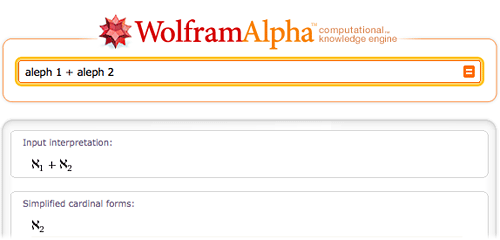In fact, multiplication and addition of transfinite cardinals is trivial—the result is just the larger of the two numbers.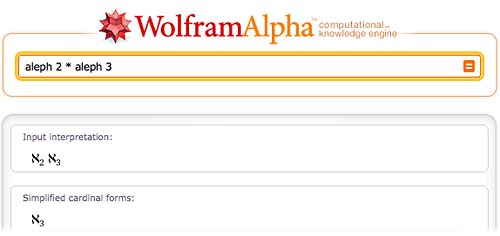Finite cardinal numbers (that is, non-negative integers) can also appear in formulas with transfinite cardinals.And transfinite arithmetic isn’t just limited to addition and multiplication. Wolfram|Alpha knows rules for manipulating exponents and factorials, too.One of the first questions that Cantor asked was the following: “Since there are more real numbers than integers, and since ℵ1 is the size of the next largest set after the set of integers, is the set of real numbers of size ℵ1?” It’s easy to prove that the set of real numbers is of size 20, so this question is equivalent to asking, “Does 20=ℵ1?”

Cantor hypothesized that the answer was “Yes,” and his conjecture has come to be known as the continuum hypothesis. (The word “continuum” is an archaic name for the set of real numbers.) It certainly is a plausible hypothesis, since an answer of “No” would imply that there exists a set whose size is intermediate between the set of integers and the set of reals, and no such set has ever been found.

In many cases, if the continuum hypothesis can be used to simplify a result in transfinite arithmetic, then Wolfram|Alpha will display a brief note to that effect and describe the simplified result.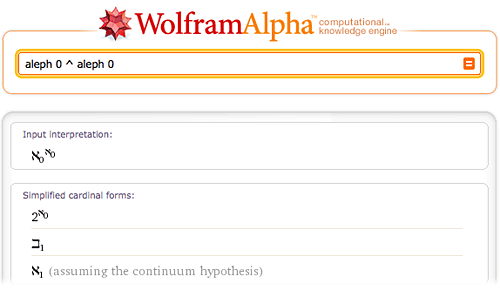Note that the cardinal number(pronounced “beth-one”) is an abbreviation for 20. And more generally, for any non-negative integer n,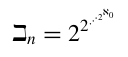is a tower of exponents containing n many 2s.

Cantor spent much effort trying to prove his continuum hypothesis, but without success. In fact, in an address to the International Congress of Mathematicians in 1900, David Hilbert listed the continuum hypothesis as one of the greatest mathematical challenges for the twentieth century. Pursuing that challenge, Kurt Gödel showed that the known axioms of mathematics are insufficient to disprove the hypothesis. This seemed tantalizingly close to a proof of the continuum hypothesis, but then in 1963, Paul Cohen showed that the known axioms are also insufficient to prove the continuum hypothesis. In other words, the known facts of mathematics are simply not sufficient to answer Cantor’s question.

During the late 1800s, it may have seemed that Cantor’s ideas would allow us to perform transfinite cardinal arithmetic just as easily as we perform ordinary finite arithmetic. But in retrospect, we can see that Cantor’s ideas only allowed us to perform cardinal arithmetic in certain specialized cases. Many simple formulas, such as 20 cannot be evaluated without significant new insights into the nature of infinity.

Cohen, P. J. Set Theory and the Continuum Hypothesis, W. A. Benjamin, Inc., 1966.

Dauben, J. W. Georg Cantor: His Mathematics and Philosophy of the Infinite, Princeton University Press, 1979.

Enderton, H. B. Elements of Set Theory, Academic Press, 1977.

Rucker, R. Infinity and the Mind: The Science and Philosophy of the Infinite, Birkhäuser, 1982.

I simply find the one-to-one point correspondence argument invalid. Let us suppose that the point is represented by a circle – similar to basic algebraic substitution. Now let us enlarge the outer circle so as to make my point clearer.

There will come a time when B would be intersected by two points – namely A and C. And as the outer-circle becomes larger and larger, we could say that point B would form a correspondence to a multitude of points – which could only be describe in numbers to be a large one. And if we continue this enlargement process, we can assume that we are getting closer and closer to infinity but never reaching it. Thus, it would seem that in the material world, we can only talk about infinity but only observe it as the limit of x as it approaches infinity, x being the number of circles or points that corresponds to B.

It seems I haven’t read the post entirely.

Posted by Ian Patrick Co September 13, 2010 at 5:45 am

Cantors view of infinity is confused. The reality is there are no points, the lines in the example are in fact segments which have a width no matter how small we try to make them! It follows from this that our so called points actually represent arcs of the circle instead of a so called dimensionless point. This also true of the centre which is a circle in itself and can never be a dimensionless point, we only consider of it as such because of the limits of our perceptions.

If we drew another line next to A such that its ‘arc’ is of the same size as that of A and such that it is immediately drawn next to A then when we draw that line back to the centre we will find that the this new line will slightly overlap with the arc on the inner circle (point B from our first line), hence we have lost our unique one-to-one mapping between the two circles and the whole argument breaks down!

N.B. Remember that the line will be of the same width all the way to the centre.

Posted by m.cleverson September 16, 2011 at 4:41 pm

If we know we can’t prove or disprove it then isn’t the only option left to us to either accept it as an axiom or take as an axiom the existence of sets with intermediate cardinalities? On another note entirely what about objects such as the set of all sets (I suppose I should say class of all sets) the set of all sets would contain the power sets of all the sets it contains which means the smallest number which could possibly be its cardinality would have to be beth-aleph 0. Instead of coming to this conclusion it seems we have come to the conclusion that the set of all sets is an ill defined object. But this seems to me to be dancing around the point since we can simply start with the set of all integers (by all accounts definitely well behaved enough to be a set) and we add to it its own power set and then repeat this operation ad infinitum (I suppose to be precise we do it aleph 0 times). We now ask what is the cardinality of the set that is the result of this operation? Clearly this set has cardinality beth-aleph 0 and furthermore must be its own power set since taking its power set one more time doesn’t change the set. So this set also violates the theorem that no set can have a one to one correspondence with its power set. Perhaps taking a power set an infinite number of times is somehow logically flawed since it leads to this contradiction. But saying that such an object created by this process is a logical aberration seems to me to be no better than the sort of vague way that people dealt with infinity before cantor.

Posted by Tim Anderton September 14, 2010 at 12:25 pm

Tim,

There have been attempts to provide new axioms that decide the continuum hypothesis. Unfortunately, there has never been a consensus that these newly-proposed axioms are true. For example, Gödel proposed the Axiom of Constructibility (also known as “V=L”), which implies that the continuum hypothesis is true. In contrast, many contemporary set theorists argue that the continuum hypothesis may be false. In particular, see http://www.ams.org/notices/200106/fea-woodin.pdf and http://www.ams.org/notices/200107/fea-woodin.pdf.

Regarding your other question, sets with cardinality beth-aleph-0 (also known as beth-omega) are commonly studied in set theory, but are not their own power sets. See the reference by Enderton that I provided in the “Further Reading” section.

You may be interested in an alternative set theory, known as Quine’s New Foundations, which allows for the existence of a set of all sets, while still avoiding Russell’s famous paradox. See Chapter 9 of Rosser’s textbook Logic for Mathematicians.

Posted by Matthew Szudzik September 15, 2010 at 3:34 pm

This implementation of cardinal arithmetic is broken.

In general, you can check that beth (i+1) = 2^(aleph i).

Computing [2 ^ (2 ^ aleph 0)] ^ (aleph 3) yields a result of uncountable.
Now since 2 ^ (2 ^ aleph 0) = beth 2, we should get the same result for beth 2 ^ aleph 3, but it outputs that beth 2 ^ aleph 3 is a countable cardinal, which is clearly nonsense, since beth 2 itself is uncountable.

For beth i ^ aleph 3, i =2,3 appear to be broken in the same way, but other i don’t seem broken.
For beth i ^ aleph 2, i=3 appears to be the only one that is broken.

Posted by Tom Cuchta September 16, 2010 at 2:31 pm

[…] mathematics capabilities. But those of you who don’t have quite as much interest in, say, transfinite cardinal arithmetic, can rest assured that we haven’t stopped adding more general pop culture data to the […]

Posted by Wolfram|Alpha Blog : Lights, Camera, Movie Data! September 17, 2010 at 9:45 am

Hi, I’ve been thinking about all this concept… and I’m leaning towards to something that we can observe.

I mean, if circles have infinite number of points, then my pencil contains infinite number of lead atoms as well. Which I think is simply not true. We can look at a point as a circle or sphere with a diameter of zero. So if we enlarge this diameter, we will find that it would not be possible to create a one-to-one correspondence.

I think it is not possible for infinity to exist in our finite universe. But it could easily substitute the concept of large set of data which are not too practical to count.

Posted by Ian Patrick Co October 11, 2010 at 2:27 am

“if circles have infinite number of points, then my pencil contains infinite number of lead atoms as well.”

There are points within and between atoms on any line and atoms aren’t continuous, therefor there is no 1-to-1 correspondence between atoms in a line and points on that line.
Although there are 5 x 10^22 atoms of carbon / gram in your pencil lead (which SEEMS infinite), there is not an infinite number of atoms there.

Posted by zweibarren May 31, 2012 at 8:39 pm

What a fascinating discourse. As a non-mathematician this is most exciting. We could try other non-computational approaches. For instance, taking the simple case of integer number infinity, we could propose that the ‘infinity’ represents a change of state or phase – a ‘realm of all integer numberness’. In other words the infinity of integer number is a dimension-like entity – a realm of quality of all integer numberness – limited by quality not by magnitude. So we have a kind of particle / field duality between a unique distinction of number, defined by magnitude, and the quality of integer numberness, which is a realm of quality of integer number and is a dimension-like entity.

From the above we may suggest that as a number approaches infinity it becomes more ‘dimension-like’ and less ‘number-like’

So it is possible, for instance, for ‘n=n squared’ to be true if it is the dimensional attribute that is expressed representing the truth of the statement and therefore is the common reality of (n-ness) on both sides of the equals sign. Or as in the case of the cardinality of concentric circles, the dimensional attribute of ‘circleness’ is common as an infinite realm of circleness for any size of concentric circular expansion of a point. (circleness + circleness = circleness)

So I suppose what I am trying to say is that maybe we can consider infinities and other mathematical functions as having computational and non-computational components which have different expression and significances as magnitudes or dimensional entities.

just a thought?

Posted by dallas simpson May 4, 2011 at 10:24 am

Ian Patrick Co said:
“I mean, if circles have infinite number of points, then my pencil contains infinite number of lead atoms as well. Which I think is simply not true. We can look at a point as a circle or sphere with a diameter of zero. So if we enlarge this diameter, we will find that it would not be possible to create a one-to-one correspondence.”

This is a profound statement. The statement above is, apparently, referring to a dimensional compactification of a circle or a sphere to a point – the ‘point’ being a potential circle or sphere in one dimension, or do mathematicians refer to a point as zero dimension?. Anyway, A point has distinction as locus, but no other extension. The issue of size or scale in Euclidean (virtual) space is different, surely, to drawing a circular line (circumference) with a physical pencil in spacetime. No written chemical equation (a virtual /mathematical concept) has ever, of itself, transformed or produced a single atom of physical substance.

But considering the case of any line we could say that ‘any line contains an infinite number of points’, and as soon as we introduce ‘infinity’ we somehow abolish ‘size’. Any line length can be mapped to any other line length. So dimensional extensions of the point to a line, circle or sphere still contains the infinity paradox of abolishing ‘size’ through a declaration of the infinity of points. Which emphasises the statement I made previously suggesting that infinities carry a dimensional, non-computation element as part of its reality.

The mapping of all concentric circles to each other to me seems to also contain an essence of the ‘particle / wave’ duality, and the attribute descriptor (name / quality of the entity) as a fundamental non-computational dimension-like aspect of the reality (circle / line / sphere) expressed in our mind.

The principle of expressed and unexpressed potentialities is relevant here.

just a few more thoughts…

Posted by dallas simpson May 6, 2011 at 2:46 am

Really part of what we are saying is that “points” have no physical size. So from a physical standpoint they are nothing. We are then saying that you can put a whole lot of “nothings” (an infinite number) on any sized physical object. This means I could shove an infinite number of “nothings” up my nose. So what? This is clearly just a mathematical reality, not a physical one.

Posted by Dao Jones March 5, 2014 at 2:21 am